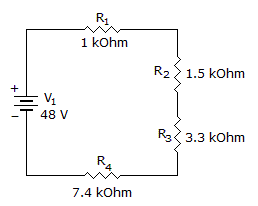# Electronics - Series Circuits

### Exercise :: Series Circuits - General Questions

16.

A short circuit has:

 A. too much resistance B. no conductance C. no resistance D. low current

Explanation:

No answer description available for this question. Let us discuss.

17.

If three resistors of 1.5 kilohms, 470 ohms, and 3300 ohms are in series with a 25-volt source, what is the total circuit current?

 A. 210 mA B. 5.2 mA C. 4.7 mA D. .007 A

Explanation:

No answer description available for this question. Let us discuss.

18.

What is the total power in the circuit?A. 170 mW B. 1.7 W C. 17 W D. 170 W

Explanation:

No answer description available for this question. Let us discuss.

19.

A string of resistors in a series circuit will:

 A. divide the source voltage in proportion to their values B. reduce the power to zero C. cause the current to divide D. increase the source voltage in proportion to the values

Explanation:

No answer description available for this question. Let us discuss.

20.While putting three 1.5 V batteries into a flashlight, you put one in backwards. The flashlight will be ______________.

 A. brighter than normal B. dimmer than normal C. off D. the same